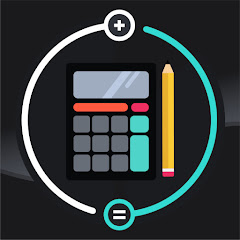# Calcuonline: All In One

1K+Everyone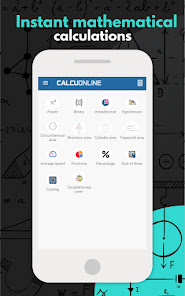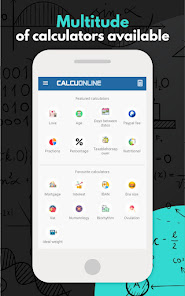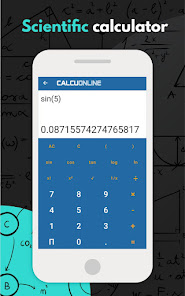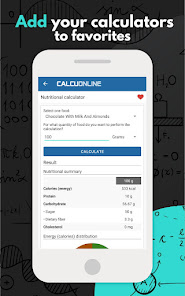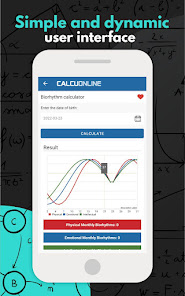We are pleased to present Calcuonline: the complete ALL IN ONE calculator for Android.

Whether you are a student or a professional, you can solve all kinds of mathematical operations - from the simplest to the most complex - in just seconds with this free calculator.

It offers more than 40 calculators covering areas such as finance, mathematical analysis, health and sports, astrology, leisure, and pregnancy.

Thus, you can perform a variety of operations from simple additions to the calculation of trigonometric values, nutritional values and PayPal fees.

Simple interface

The scientific calculator is not only accurate in its results, but also has a simple and friendly interface to enhance user experience.

All calculators and converters are organized into their respective categories, and you can switch from one type to another with a single tap of the screen.

Financial

Mortgage calculator
Interest calculator
IBAN calculator
ROI calculator
Discount calculator
VAT calculator
PayPal commission calculator

Mathematical analysis

Power calculator
Binary calculator
Hypotenuse calculator
Circumference area calculator
Rhombus area calculator
Cylinder area calculator
Trapezoid area calculator
Average apeed calculator
Fractions calculator
Percentage calculator
Rule of three calculator
Cooling calculator
Taxablehorsepower calculator

Health and Sports

Estimated maximum heart rate calculator
Bmr calculator
BMI calculator
Ideal weight calculator
Body fat calculator
Nutritional calculator

Astrological

Love calculator
Numerology calculator
Biorhythm calculator
Chinese zodiac sign calculator
Ascendant calculator

Leisure

Age calculator
Days between dates calculator
Bra size calculator
Bike size calculator

Pregnancy

Pregnancy due date calculator
Baby gender calculator
Ovulation calculator
Weeks of pregnancy to months calculator

Scientific calculator for everyone

The all-in-one calculator, Calcuonline, is not only the perfect tool for students or professionals in engineering, mathematics, economics and accounting, but is also ideal for those who do not require such complex calculations or just want to have fun calculating compatibility with another person or someone's age.

Try it now, it's free

Would you believe us if we told you that you don't have to pay anything for this super calculator? That's right, it is a free calculator that you can try right now to make all the calculations you want.
Updated on
Mar 29, 2022

## Data safety